### Magnetic Effects Of Electric Currents, And Electrical Measurements

Chapter XII. Magnetic Effects Of Electric Currents,And Electrical Measurements.

### (1) The Magnetic Effect of Electric Currents

255. The Magnetic Effect.?Of all the effects of electric currents, it is generally conceded that the magnetic effect is the one of greatest practical importance, and it is also the one most extensively used. An experiment illustrating this effect has been described in Art. 239. This experiment shows that an electric current, if parallel to a magnetic needle, and near it will deflect the north-seeking pole of the needle to the right or left depending upon the direction of the current flow. This deflection of the magnetic needle is due to the fact that surrounding every electric current are magnetic lines of force. It is this magnetic field of the current that causes the needle to turn. The position taken by the needle is the resultant of the forces of two magnetic fields; one, the earth's field, the other, that of the current.
256. Right-hand Rule for a Conductor.?To show the presence of the magnetic field about a current, pass a thick copper wire vertically through a sheet of paper, and connect the ends of the wire to a source of current. While the current (this should be as much as 10 amperes if possible) is flowing, sprinkle iron filings upon the paper and tap gently. The filings will arrange themselves in circles about the wire showing the magnetic field. (See Fig. 229.) The needle of a magnetoscope tends to place itself parallel to the lines of force of this field and from this action or[Pg 280] tendency the direction of the magnetic lines about a current may be determined. The following rule is helpful and should be memorized: Grasp the conductor with the right hand with the outstretched thumb in the direction that the current is flowing. The fingers will then encircle the wire in the direction of the lines of force. This rule may be reversed, for, if the fingers of the right hand grasp the wire so as to point with the magnetic field, then the current flows in the direction in which the thumb points. (See Fig. 230.)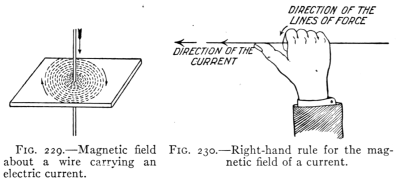Fig. 229.?Magnetic field about a wire carrying an electric current.
Fig. 230.?Right-hand rule for the magnetic field of a current.
257. Magnetic Field of a Helix.?If a wire be wound about a cylinder to form a cylindrical coil with parallel turns, it forms a helix or solenoid. The shape of the magnetic field about a current depends upon the form of the conductor. If the latter is in the form of a helix its magnetic field resembles that of a straight bar magnet. (See Fig. 231). In fact the helix has the properties of a magnet with north- and south-seeking poles while a current is flowing through it. If such a coil is suspended so as to turn freely, it tends to turn until the field within it is parallel to the earth's magnetic field. Such a suspended helix may therefore be used as a compass. In order to strengthen the magnetic field of a helix or solenoid, the space within its turns is filled with iron, often in the form[Pg 281] of small soft-iron wires. This bundle of iron wire is called the core of the helix. The core becomes strongly magnetized by the field of the helix while the current is flowing and quickly loses its magnetic force when the current is stopped. The direction of the current in a helix (Fig. 232) or the polarity of its core may be determined by another right-hand rule. If the helix is grasped with the right hand so that the fingers point in the direction in which the current is flowing, the extended thumb will point in the direction of the north pole of the helix. On the other hand, if the poles of the helix are known, then, when the helix is grasped with the right hand so that the thumb points to the north-seeking pole, the current is flowing in the wires in the direction that the fingers point.Fig. 231.?The magnetic field of a helix.
Fig. 232.?Right-hand rule for a helix.
258. The Electromagnet.?These "right-hand" rules are applied in many different devices. Among these, perhaps the most important is the electromagnet, which is used in the electric bell, the telegraph, the telephone, the dynamo, the motor, and many other electric contrivances.
The electromagnet is defined as a mass of iron around which is placed a helix for conducting an electric current. On account of its large permeability, the iron core of the helix adds greatly to the effectiveness of the electromagnet, since the magnetism of the iron is added to that of the current in the helix. The magnetism remaining[Pg 282] in the iron after the current stops is called the residual magnetism. The residual magnetism is small when the core is made of small wires or thin plates, but is larger when the iron core is solid. Like artificial steel magnets, electromagnets are usually of two forms, bar and horseshoe. (See Figs. 233 and 234.) For most purposes the horseshoe form is the more effective since it permits a complete iron circuit for the magnetic lines of force. (See Fig. 235.) This is the form used in the electric bell, in the telegraph sounder, and in lifting magnets. (See Fig. 236.)Fig. 233.?A bar electromagnet.
Fig. 234.?A horseshoe electromagnet.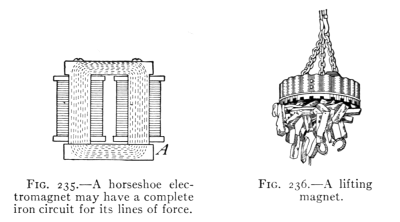Fig. 235.?A horseshoe electromagnet may have a complete iron circuit for its lines of force.
Fig. 236.?A lifting magnet.
259. Effective Electromagnets.?The magnetic effect of a current in a helix is small, hence the force usually is increased by inserting a core of iron. When at first man[Pg 283] tried to signal with electromagnets at a distance it was found that the current would not work the electromagnet. An American by the name of Joseph Henry discovered the remedy for this condition. He found that if the copper wire was insulated by wrapping silk thread about it, and then many layers of the silk insulated wire were wound upon a spool with an iron core, that the magnet would work at a great distance from the source of current. If the current is increased, the magnet is stronger than at first. Thus an electromagnet may be made stronger by (a) increasing the number of turns of wire in its coils and by (b) sending a stronger current through it.Fig. 237.?A simple telegraph circuit.
260. The Telegraph.?The invention of an effective electromagnet by Henry made possible the electric telegraph. In its simplest form it consists of a battery, C, a key, K, and a sounder, S, with connecting wires. (See Fig. 237.) The sounder (Fig. 238) contains a horseshoe electromagnet and a bar of soft iron across its poles called an armature, A, attached to a lever L. When the key is closed, the electromagnet draws down the armature and lever until the latter hits a stop O, making a click. When the key is raised, the magnet releases the armature which is raised by the action of a spring at S until the lever hits a stop at T making another click. Closing and opening the circuit at K will start and stop the current which operates S which may be 100 miles or more from K. One voltaic cell will work a sounder in the same room. But if many miles of wire are in the circuit, the E.M.F. of a single cell will not force sufficient current through the long wire to operate the sounder.
[Pg 284]Fig. 238.?A telegraph sounder.Fig. 239.?A telegraph relay.
Fig. 240.?How the relay is used.Samuel F. B. Morse (1791-1872). Inventor of the electromagnetic recording telegraph and of the dot and dash alphabet.

Samuel F. B. Morse
"From Appleton's Cyclopedia of American Biography, Copyright 1888 by D. Appleton & Co."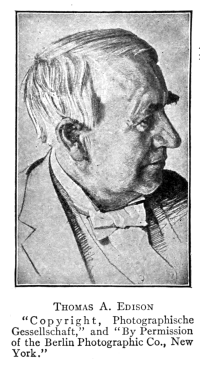Thomas A. Edison, Orange, New Jersey. Invented the incandescent lamp; phonograph; moving picture; most noted inventor of electrical appliances of the present day.

Thomas A. Edison
"Copyright, Photographische Gessellschaft," and "By Permission of the Berlin Photographic Co., New York."
A battery of several cells is then required. Even a large battery is insufficient to operate a long line containing many sounders in circuit. Recourse is therefore usually made to a more sensitive device called a relay. (See Fig. 239.) In the relay a very small current will magnetize its electromagnet enough to draw toward it the delicately hung armature thereby closing a second circuit which contains a sounder and a battery. (See Fig. 240.) when the current in the main circuit is stopped, the armature of the relay is drawn back by a light spring. This opens the local circuit. Thus the local circuit is closed and opened by the relay just in time with the starting and stopping of the current in the main line. It is thus possible for a small current in the main line by the use of a relay, to close and open a second local circuit containing a local battery and sounder. Modern telegraph lines are operated in this manner.Fig. 241.?An electric bell and its circuit.
261. The electric bell (see Fig. 241), consists of an electromagnet, M, a soft iron armature, A, attached to the tapper, T, and a post, R. When no current is flowing a spring at S holds the armature against the post R. When current flows through the helix, its core becomes magnetized and attracts the armature, drawing it away from the post, R, and causing the tapper to hit the bell. Drawing A away from the post, however, breaks the circuit at R and the current stops. The magnetism in the core disappears releasing the armature, which is then pulled back by the spring S against the post R. This completes the circuit and the process repeats itself several times a second as long as the current flows.Fig. 242.?Magnetizing by the discharge of a Leyden jar.
262. Static and Current Electricity Compared.?The likeness between a discharge of static electricity and an electric current may be shown by winding a coil of insulated wire about a glass tube which contains a steel needle. If a Leyden jar (see Fig. 242) is discharged through the coil the steel needle is usually found to be magnetized, showing that the discharge of the static electricity has a magnetic effect similar to that of an electric current. Sometimes a given end of the needle has a north pole and at other times a south pole. This is believed to indicate[Pg 288] that the charge of the Leyden jar is oscillatory, and that in different discharges sometimes a surge in one direction and at other times a surge in the reverse direction has been most effective in magnetizing the needle. Compare this action with that described in Art. 233.

#### Important Topics

1. Right-hand rules, for conductor, for helix.
2. The electromagnet, two forms, where used?
3. Likeness between static and current electricity.
4. The electric bell, parts, action.
5. The telegraph, key, sounder, relay.

#### Exercises

1. What is the difference between an electric charge and a current?
2. How can a magnetic effect be produced from an electric charge?
3. What is a magnetic field? Give two evidences of a magnetic field about a current in a wire?
4. A current is flowing north in trolley wire, what is the direction of the magnetic field under the wire? Explain.
5. What would be the result if a hard steel core were placed in the electromagnet? Explain.
6. If the north-seeking pole of a helix is facing you, does the current in the coils before you move in a clockwise or in a counter-clockwise direction? Explain.
7. A helix is placed horizontally with its north-seeking pole toward the north. Does the current in the wire at the top of the helix move east or west? Explain.
8. State at least six conditions any one of which will put an electric bell circuit out of commission.
9. If one desires to insert a battery into a telegraph circuit already in operation, how will he determine the direction of the current in the wire?
10. If a boy who had magnetized his knife blade in a physics laboratory, pointed end south-seeking, should lose his way in the woods on a cloudy day, how could he determine his way out?
11. At a certain point the earth's field acts north, that of an electric current, east. The magnetoscope needle points exactly northeast when placed at that point. How do the two magnetic fields compare?
[Pg 289]

### (2) Electrical Measurements

263. Galvanometers.?In using electric currents it is often necessary or desirable to be able to know not only that a given current is weak or strong, but precisely what its strength is. We can determine the relative strengths of two currents by the use of a galvanometer.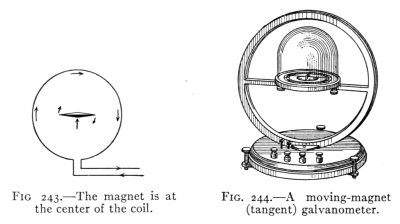Fig. 243.?The magnet is at the center of the coil.
Fig. 244.?A moving-magnet (tangent) galvanometer.
The older or moving-magnet type of galvanometer is similar to the galvanoscope mentioned in Art. 239. It consists of a magnetic needle mounted at the center of a coil of wire. The coil is placed facing east and west, so that the needle will be held by the earth's magnetic field parallel to the plane of the coil. When a current is sent through the coil a magnetic field is produced within it. This deflects the needle, its north end turning east or west depending upon the direction of the current. (See Fig. 243.) The coils of a moving-magnet or tangent galvanometer (see Fig. 244) are large and firmly fastened to the base, while the magnet is small.
The moving-coil type of galvanometer (see Fig. 245) consists of a large magnet fastened to the frame of the device. The magnet usually has a horseshoe form to[Pg 290] produce as strong a field as possible. The coil is wound on a light rectangular frame and is suspended between the two poles of the magnet. To concentrate the magnetic field, a cylinder of soft iron is usually placed within the coil. Fig. 246 represents a common form of moving-coil galvanometer.Fig. 245.?To illustrate the principle of the moving-coil galvanometer.
Fig. 246.?A moving-coil (D'Arsonval) galvanometer.
264. Measurement of Electric Currents.?A galvanometer enables one to compare electric currents. To measure electric currents it is necessary to employ a unit of electrical quantity, just as in measuring the quantity of water delivered by a pipe, a unit of liquid measure is employed; thus, e.g., the current delivered by a given pipe may be 2 gallons of water per second, so in measuring the flow of an electric current one may speak of two coulombs per second. The coulomb is the unit quantity of electricity just as the unit of quantity of water is the gallon.
For most practical purposes, however, we are more interested in the rate or intensity of flow of current than in[Pg 291] the actual quantity delivered. The unit of rate of flow or current is called the ampere.
In determining the exact quantity of an electric current, physicists make use of a device called a coulomb meter. (See Fig. 247.) This contains a solution of silver nitrate in which are placed two silver plates. The current to be measured is sent through the solution, in at one plate and out at the other. The plate where the current goes in, the anode, A (Fig. 247), loses in weight since some of the silver is dissolved. The plate where the current goes out, the cathode, C, increases in weight since some of the silver is deposited. By an international agreement, the intensity of the current which deposits silver at the rate of 0.001118 g. per second is 1 ampere. This is equal to 4.025 g. per hour.Fig. 247.?A coulomb meter, the anode A is separated from the cathode C by a porous cup.
The coulomb is defined as the quantity of electricity delivered by a current of one ampere during one second.
A 40-watt-incandescent lamp takes about 0.4 ampere of current. An arc lamp takes from 6 to 15 amperes. A new dry cell may send 20 amperes through a testing meter. A street car may take from 50 to 100 amperes.
265. The Ammeter.?The method described above is not used ordinarily for measuring current strengths on account of its inconvenience. The usual device employed is an ammeter. This instrument is a moving-coil galvanometer.[Pg 292] It contains, wound on a light form, a coil of fine copper wire. The form is mounted on jewel bearings between the poles of a strong permanent horseshoe magnet. (See Fig. 248.) As in other moving-coil galvanometers, a soft iron cylinder within the form concentrates the field of the magnet. The form and its coil is held in balance by two spiral springs which also conduct current into and out of the coil.
Only a small part of the whole current measured, in some cases only 0.0001 passes through the coil, the larger part of the current passing through a metal wire or strip called a shunt[L] (see Fig. 248) connecting the binding posts of the instrument. A fixed fraction of the whole current flows through the coil. Its field crossing the field of the horseshoe magnet, tends to turn until its turning force is balanced by the spiral springs. As the coil turns it moves a pointer attached to it across a scale graduated to indicate the number of amperes in the whole current.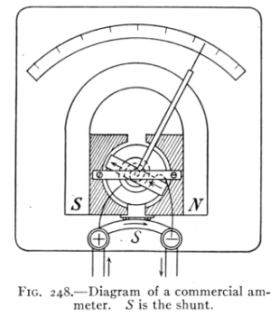Fig. 248.?Diagram of a commercial ammeter. S is the shunt.
It should be noted that while all of the current measured passed through the ammeter, but a small part goes through the coil.
[Pg 293]
266. Resistance of Conductors.?With an ammeter one may study the change produced in the amount of current flowing in a wire when a change is made in the wire conducting the current. For example, if one measures with an ammeter the current flowing from a dry cell through a long and then through a short piece of fine copper wire, it will be seen that less current flows when the long piece is used. That is, the long wire seems to hinder or to resist the passing of the current more than the short piece. In other words, the long wire is said to have more resistance.
The resistance of a conducting body is affected by several conditions.
(a) It is directly proportional to the length of the conductor, one hundred feet of wire having twice the resistance of fifty feet.
(b) It is inversely proportional to the square of the diameter; a wire 0.1 inch in diameter has four times the resistance of a wire 0.2 inch in diameter.
(c) It differs with different substances, iron having about six times as much as copper.
(d) It varies with the temperature, metals having greater resistance at a higher temperature.
Since silver is the best conductor known, the resistances of other substances are compared with it as a standard.
The ratio of the resistance of a wire of any substance as compared to the resistance of a silver wire of exactly the same diameter and length is called its relative resistance.
Purified substances arranged in order of increasing resistance for the same length and sectional area (Ayrton-Mather) are given on p. 294.
[Pg 294]
 Silver annealed 1 Copper annealed from 1.04 (Copper annealed) to 1.09 Aluminum annealed 1.64 Nickel annealed 4.69 Platinum annealed 6.09 Iron annealed 6.56 German Silver from 12.8 (German Silver) to 20.2 Mercury 63.3 Nichrome 67.5 Carbon from 2700 (Carbon) to 6700
267. The ohm, the unit of resistance, is defined by international agreement as follows: An ohm is the resistance of a column of pure mercury, 106.3 cm. long with a cross-section of a square millimeter and at a temperature of 0?C.
It should be noted that each of the four conditions affecting resistance is mentioned in the definition, viz., length, cross-section, material, and temperature. Since it is inconvenient to handle mercury, standard resistance coils, made of an alloy of high resistance are used in comparing and measuring resistances.
A piece of copper wire No. 22 (diameter 0.644 mm.) 60. 5 ft. long has a resistance of 1 ohm. See table p. 296.
The resistance of some telephone receivers is 75 ohms, of a telegraph sounder, 4 ohms, of a relay 200 ohms.
268. Resistance of Circuits.?Every part of an electrical circuit possesses resistance. In an electric-bell circuit, for instance, the wires, the bell, the push-button, and the cell itself, each offers a definite resistance to the passage of the current. The resistance within the cell is termed internal resistance, while the resistance of the parts outside of the electric generator is called external resistance.
[Pg 295]
269. Electromotive Force.?In order to set in motion anything, some force must be applied. This is as true of electricity as of solids, liquids, or gases. By analogy that which is exerted by a battery or by a dynamo in causing current to flow is called an electromotive force. The unit of electromotive force, the volt, may be defined as the electromotive force that will drive a current of 1 ampere through the resistance of 1 ohm. The electromotive force of a dry cell is about 1.5 volts, of a Daniell cell 1.08 volts. Most electric light circuits in buildings carry current at 110 or 220 volts pressure. Currents for street cars have an electromotive force of from 550 to 660 volts.Fig. 249.?Diagram of a commercial voltmeter.
270. The Voltmeter.?An instrument for measuring the electromotive force of electric currents is called a voltmeter (Fig. 249). It is usually a moving-coil galvanometer, and is always of high resistance. It is like an ammeter in construction and appearance. In fact, a voltmeter is an ammeter which has had its shunt removed or disconnected. In place of a shunt, the voltmeter uses a coil of wire of high resistance (see R, Fig. 249) in series with the galvanometer coil. The high resistance of the voltmeter permits but a very small current to flow through it. Hence a voltmeter must be placed across a circuit and[Pg 296] not in it. In other words a voltmeter is connected in shunt, while an ammeter is in series with the circuit as is shown in Fig. 250.
Dimensions and Functions of Copper Wires
 B. & S. gauge number Diameter Circular mils Sectional area in square millimeters Weight and length, Density = 8.9, feet per pound Resistance at 24?C., feet per ohm Capacity in amperes Mils Millimeters 0000 460.000 11.684 211,600.00 107.219 1.56 19,929.700 312.0 000 409.640 10.405 167,805.00 85.028 1.97 15,804.900 262.0 00 364.800 9.266 133,079.40 67.431 2.49 12,534.200 220.0 0 324.950 8.254 105,592.50 53.470 3.13 9,945.300 185.0 2 257.630 6.544 66,373.00 33.631 4.99 6,251.400 131.0 4 204.310 5.189 41,742.00 21.151 7.93 3,931.600 92.3 6 162.020 4.115 26,250.50 13.301 12.61 2,472.400 65.2 8 128.490 3.264 16,509.00 8.366 20.05 1,555.000 46.1 10 101.890 2.588 10,381.00 5.260 31.38 977.800 32.5 12 80.808 2.053 6,529.90 3.309 50.69 615.020 23.0 14 64.084 1.628 4,106.80 2.081 80.59 386.800 16.2 16 50.820 1.291 2,582.90 1.309 128.14 243.250 11.5 18 40.303 1.024 1,624.30 0.823 203.76 152.990 8.1 20 31.961 0.812 1,021.50 0.5176 324.00 96.210 5.7 22 25.347 0.644 642.70 0.3255 515.15 60.510 4.0 24 20.100 0.511 504.01 0.2047 819.21 38.050 2.8 26 15.940 0.405 254.01 0.1288 1,302.61 23.930 2.0 28 12.641 0.321 159.79 0.08097 2,071.22 15.050 1.4 30 10.025 0.255 100.50 0.05092 3,293.97 9.466 1.0 32 7.950 0.202 63.20 0.03203 5,236.66 5.952 0.70 34 6.304 0.160 39.74 0.02014 8,328.30 3.743 0.50 36 5.000 0.127 25.00 0.01267 13,238.83 2.355 0.35 38 3.965 0.101 15.72 0.00797 20,854.65 1.481 0.25 40 3.144 0.080 9.89 0.00501 33,175.94 0.931 0.17

#### Important Topics

(1) Galvanometers: (1) moving magnet, fixed coil; (2) moving coil, fixed magnet, ammeter, voltmeter.
(2) Unit of quantity, coulomb.
(3) Unit of current, ampere.
(4) Unit of resistance, ohm.
(5) Unit of electromotive force, volt.
[Pg 297]

#### Exercises

1. How will the resistance of 20 ft. of No. 22 German silver wire compare with that of 10 ft. of No. 22 copper wire? Explain.
2. Where in a circuit is copper wire desirable? Where should German silver wire be used?
3. Explain the action of the ammeter. Why does not the needle or coil swing the full distance with a small current?
4. Why is a telegraph sounder more apt to work on a short line than upon a long one?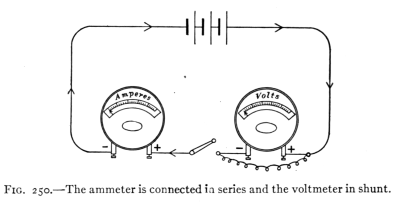Fig. 250.?The ammeter is connected in series and the voltmeter in shunt.
5. Find the resistance of 15 miles of copper telephone wire No. 12. (See table p. 296.)
6. What will be the weight and resistance of 1,000 feet of No. 20 copper wire?
7. A storage battery sends 4 amperes of current through a plating solution. How much silver will it deposit in 2 hours?
8. (a) Compare the diameters of No. 22 and No. 16 copper wire.
(b) Compare the lengths of the same wires giving 1 ohm resistance.
(c) What relation exists between (a) and (b)?
9. Why is an electric bell circuit usually open while a telegraph line circuit is usually closed?
10. A copper wire and an iron wire of the same length are found to have the same resistance. Which is thicker? Why?
11. Why are electric bells usually arranged in parallel instead of in series?
12. What would happen if a voltmeter were put in series in a line?
[Pg 298]

### (3) Ohm's Law and Electrical Circuits

271. Conditions Affecting Current Flow.?Sometimes over a long circuit one cell will not work a telegraph sounder. In such a case, two, three, or more cells are connected so that the zinc of one is joined to the copper plate of the other. When connected in this way the cells are said to be in series (Fig. 251). In the figure A represents a voltmeter. It is found that when cells are in series the E.M.F. of the battery is the sum of the electromotive forces of the cells. An ammeter in the circuit shows increased current as the cells are added. Hence if the resistance of the circuit remains unchanged, the greater the E.M.F. the greater is the current strength. In this respect, the movement of electricity in a circuit is similar to the flow of water in a small pipe under pressure, as in the latter the flow of water increases as the pressure becomes greater. The current in a circuit may also be increased by lessening the resistance, since the current through a long wire is less than that through a short one, just as the flow of water will be greater through a short pipe than through a long one. To increase the current flowing in an electric circuit, one may therefore either increase the E.M.F. or decrease the resistance.Fig. 251.?Diagram of cells connected in series.
272. Ohm's Law.?The relation between the electromotive force applied to a circuit, its resistance, and the current produced was discovered in 1827 by George Ohm. Ohm's law, one of the most important laws of electricity, states that, in any circuit, the current in amperes equals the electromotive force in volts divided by the resistance in ohms.
[Pg 299]
This principle is usually expressed thus:
Current intensity = electromotive force/resistance or

Amperes = volts/ohms or I = E/RFig. 252.?The street cars are connected in parallel with each other.
273. Resistance of Conductors in Series.?A study of the resistance of conductors when alone and when grouped in various ways is of importance since, the current flow through any circuit is dependent upon its resistance. The two most common methods of combining several conductors in a circuit are in series and in parallel. Conductors are in series when all of the current passes through each of the conductors in turn (Fig. 218), thus the cell, push-button, wires, and electric bell in an electric-bell circuit are in series. Conductors are in parallel when they are so connected that they are side by side and a part of the whole current goes through each. None of the current that passes through one conductor can go through the conductors in parallel with it. Thus the electric street cars are in parallel with each other. (See Fig. 252.) It is easily seen that none of the current passing through one car can go through any of the others. When the conductors are in series the combined resistance is the sum of the several resistances. Thus in an electric-bell circuit if the battery has a resistance of 1 ohm, the bell of 2 ohms, and the wire 1 ohm, the total resistance in the circuit is 4 ohms. When conductors are in parallel the combined resistance is always less than the separate resistances. Just as a crowd of people meets less resistance in leaving a building through several exits, so electricity[Pg 300] finds less resistance in moving from one point to another along several parallel lines, than along one of the lines.
274. Resistance of Conductors in Parallel.?If three conductors of equal resistance are in parallel, the combined resistance is just one-third the resistance of each separately (Fig. 253). The rule that states the relation between the combined resistance of conductors in parallel and the separate resistances is as follows:The combined resistance of conductors in parallel is the reciprocal of the sum of the reciprocals of the several resistances. For example, find the combined resistance of three unequal resistances in parallel; the first being 4 ohms, the second, 6 ohms, and third 3 ohms. The reciprocals of the three resistances are 1/4, 1/6, and 1/3. Their sum equals 6/24 + 4/24 + 8/24 = 18/24. The reciprocal of this is 24/18 which equals 1-1/3 ohms, the combined resistance.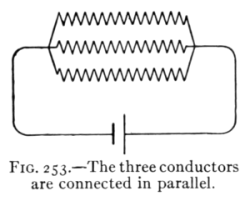Fig. 253.?The three conductors are connected in parallel.
This rule may be understood better if we consider the conductance of the conductors in parallel. Since the conductance of a two ohm wire is just one-half that of a one-ohm wire, we say that the conductance of a body is inversely as the resistance, or that it is the reciprocal of the resistance. The conductance of the 4-, 6-, and 3-ohm coils will therefore be respectively 1/4, 1/6, and 1/3, and since the combined conductance is the sum of the several conductances, the total conductance is 18/24. Also since this is the reciprocal of the total resistance, the latter is 24/18 or 1-1/3 ohms.
When two or more conductors are connected in parallel each one is said to be a shunt of the others. Many circuits are connected in shunt or in parallel. Fig. 254 represents four lamps in parallel. Incandescent lamps in buildings are usually connected in parallel, while arc lamps are[Pg 301] usually connected in series. Fig. 255 represents four lamps in series.

#### Important Topics

1. Conditions affecting current flow, (a) E.M.F., (b) resistance.
2. Ohm's law, three forms for formula.
3. Resistance of conductors: (a) in series, (b) in parallel; how computed, illustrations.Fig. 255.?The four lamps are connected in series.
Fig. 254.?The four lamps are connected in parallel.

#### Exercises

1. What current flows through a circuit if its E.M.F. is 110 volts and the resistance is 220 ohms?
2. A circuit contains four conductors in series with resistances of 10, 15, 6, and 9 ohms respectively. What current will flow through this circuit at 110 volts pressure? What will be the resistance of these four conductors in parallel?
3. What is the combined resistance of 8 conductors in parallel if each is 220 ohms? What current will flow through these 8 conductors at 110 volts pressure?
4. What is the resistance of a circuit carrying 22 amperes, if the E.M.F. is 20 volts?
5. What E.M.F. will send 8 amperes of current through a circuit of 75 ohms resistance?
6. How does the voltmeter differ from the ammeter?
7. How can one determine the resistance of a conductor?
8. The resistance of a hot incandescent lamp is 100 ohms. The current used is 1.1 amperes. Find the E.M.F. applied.
9. What is the resistance of the wires in an electric heater if the current used is 10 amperes, the voltage being 110?
10. The resistance of 1000 ft. of No. 36 copper wire is 424 ohms. How many feet should be used in winding a 200 ohms relay?
11. The resistance of No. 00 trolley wire is 0.80 ohm per 1000 ft. What is the resistance of a line 1 mile long?
12. A wire has a resistance of 20 ohms. It is joined in parallel with another wire of 6 ohms, find their combined resistance.
[Pg 302]
13. The separate resistances of two incandescent lamps are 200 ohms and 70 ohms. What is their combined resistance when joined in parallel? When joined in series?

### (4) Methods of Grouping Cells and Measuring Resistance

275. Internal Resistance of a Voltaic Cell.?The current produced by a voltaic cell is affected by the resistance that the current meets in passing from one plate to another through the liquid of the cell. This is called the internal resistance of the cell. A Daniell cell has several (1-5) ohms internal resistance. The resistance of dry cells varies from less than 0.1 of an ohm when new to several ohms when old. If cells are joined together their combined internal resistance depends upon the method of grouping the cells.Fig. 256.?The four cans exert four times the water pressure that one can will exert.
276. Cells Grouped in Series and in Parallel.?When in series the copper or carbon plate of one cell is joined to the zinc of another and so on. (See Fig. 251.) The effect of connecting, say four cells, in series may be illustrated by taking four cans of water, placed one above another. (See Fig. 256.) The combined water pressure of the series is the sum of the several pressures of the cans of water, while the opposition offered to the movement of a quantity of water through the group of cans is the sum of the several resistances of the cans. In applying this illustration to the voltaic cell, we make[Pg 303] use of Ohm's law. Let E represent the e.m.f. of a single cell, r the internal resistance of the cell, and R the external resistance or the resistance of the rest of the circuit. Consider a group of cells in series. If n represents the number of cells in series, then Ohm's law becomes
I = nE/(nr + R).

Cells are grouped in series when large E.M.F. is required to force a current through a large external resistance such as through a long telegraph line. Cells are connected in parallel when it is desired to send a large current through a small external resistance. To connect cells in parallel all the copper plates are joined and also all the zinc plates. (See Fig. 257.) To illustrate the effect of this mode of grouping cells, suppose several cans of water are placed side by side (Fig. 258). It is easily seen that the pressure of the group is the same as that of a single cell, while the resistance to the flow is less than that of a single cell. Applying this reasoning to the electric circuit we have by Ohm's law the formula for the current flow of a group of
n cells arranged in parallel I = E/((r/n) + R).Fig. 257.?Four cells connected in parallel.
Fig. 258.?The water pressure of the group in parallel is the same as that of one.
[Pg 304]
277. Illustrative Problems.?Suppose that four cells are grouped in parallel, each with an E.M.F. of 1.5 volts and an internal resistance of 2 ohms. What current will flow in the circuit if the external resistance is 2.5 ohms? Substitute in the formula for cells in parallel the values given above, and we have I = 1.5/(0.5 + 2.5) = 1.5/3 = 0.5 ampere. Suppose again that these four cells were grouped in series with the same external resistance, substituting the values in the formula for cells in series we have I = 4(1.5)/(4 ? 2 + 2.5) = 6/10.5 = 0.57 ampere.
278. Volt-ammeter Method for Finding Resistance.?Measurements of the resistance of conductors are often made. One of these methods depends upon an application of Ohm's law. It is called the volt-ammeter method since it employs both a voltmeter and an ammeter. If the conductor whose resistance is to be measured is made a part of an electric circuit, being connected in series with the ammeter and in shunt with the voltmeter, the resistance may easily be determined, since R = E/I. (See Fig. 250.) If, for example, the difference in E.M.F., or as it is often called, the fall of potential between the ends of the wire as read on the voltmeter is 2 volts, and the current is 0.5 ampere, then the resistance of the wire is 4 ohms. This method may be readily applied to find the resistance of any wire that is a part of an electric circuit.
279. The Wheatstone Bridge.?To find the resistance of a separate wire or of an electrical device another method devised by an Englishman named Wheatstone is commonly employed. This method requires that three known resistances, a, b, c, in addition to the unknown resistance[Pg 305] x be taken. These four resistances are arranged in the form of a parallelogram. (See Fig. 259.) A voltaic cell is joined to the parallelogram at the extremities of one diagonal while a moving-coil galvanometer is connected across the extremities of the other diagonal. The known resistances are changed until when on pressing the keys at E and K no current flows through the galvanometer. when this condition is reached, the four resistances form a true proportion, thus a: b = c: x.
Since the values of a, b, and c are known, x is readily computed. Thus if a = 10, b = 100, and c = 1.8 ohms, then x, the unknown resistance, equals 18 ohms, since 10: 100 = 1.8: 18. This method devised by Wheatstone may be employed to find the resistance of a great variety of objects. It is the one most commonly employed by scientists and practical electricians.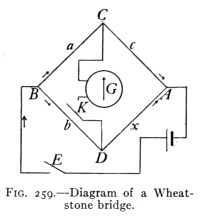Fig. 259.?Diagram of a Wheatstone bridge.

#### Important Topics

1. The internal resistance of voltaic cells.
2. Ohm's law applied to groups of cells. (a) Cells in series, (b) cells in parallel.
3. Measurement of resistance: (a) volt-ammeter method, (b) Wheatstone bridge method.

#### Exercises

1. What is the resistance of an electric bell circuit where the E.M.F. is 3 volts and the current is 0.6 ampere?
2. A telegraph wire is broken somewhere, the ends lying upon damp ground. If an E.M.F. of 30 volts is applied from the ground[Pg 306] to the wire and a current of 0.1 of an ampere flows, what is the resistance of the part connected to the ammeter. (The earth which completes the circuit from the end of the wire has very small resistance.) Why?
3. How far away is the break in the wire if the latter has a resistance of 80 ohms to the mile? Diagram.
4. What current will flow through a bell circuit of 8 ohms resistance if it contains three cells in series each with an E.M.F. of 1.5 volts and an internal resistance of 1/3 ohm?
5. If the same three cells are connected in parallel on the same circuit what current flows? Is the current in problem 4 or 5 the larger? Why?
6. If four cells each with 1.5 volts E.M.F. and an internal resistance of 0.4 ohm are connected with a circuit having an external resistance of 0.8 ohm, what current will the parallel connection give? The series connection? Which gives the larger current? Why?
7. Four Daniell cells each having 1 volt E.M.F. and 3 ohms internal resistance are connected in series with 2 telegraph sounders of 4 ohms each. The connecting wires have 6 ohms resistance. Find the current intensity.
8. A battery of 2 cells arranged in series is used to ring a door bell. The E.M.F. of each cell is 1.5 volts, internal resistance 0.3 ohm, and the resistance of the bell is 4 ohms. What is the current in amperes?
9. In the above problem find the current if the cells are connected in parallel.